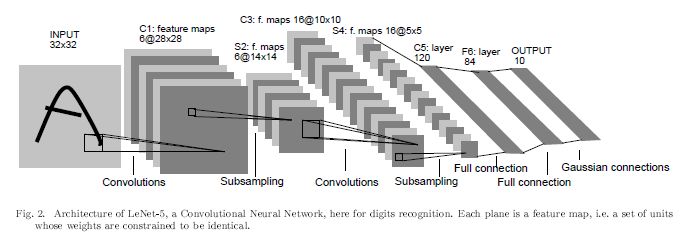# Calculating Parameters of Convolutional and Fully Connected Layers with Keras

Original article was published by Yan Ding on Deep Learning on Medium# Calculating Parameters of Convolutional and Fully Connected Layers with Keras

## Explain how to calculate the number of params and output shape of convolutional and pooling layers

When we build a model of deep learning, we always use a convolutional layer followed by a pooling layer and several fully-connected layers. It is necessary to know how many parameters in our model as well as the output shape of each layer. Let’s first see LeNet-5 which a classic architecture of the convolutional neural network.

The input shape is (32,32,3). The first layer is the convolutional layer, the kernel size is (5,5), the number of filters is 8. Followed by a max-pooling layer with kernel size (2,2) and stride is 2. The second layer is another convolutional layer, the kernel size is (5,5), the number of filters is 16. Followed by a max-pooling layer with kernel size (2,2) and stride is 2. The third layer is a fully-connected layer with 120 units. The fourth layer is a fully-connected layer with 84 units. The output layer is a softmax layer with 10 outputs.

Now let’s build this model in Keras.

`from tensorflow.keras import Sequentialfrom tensorflow.keras import layersmodel = Sequential()model.add(layers.Conv2D(8,(5,5),activation='relu',input_shape=(32,32,3)))model.add(layers.MaxPooling2D((2,2),strides=2))model.add(layers.Conv2D(16, (5,5),activation='relu'))model.add(layers.MaxPooling2D((2,2),strides=2))model.add(layers.Flatten())model.add(layers.Dense(120, activation='relu'))model.add(layers.Dense(84, activation='relu'))model.add(layers.Dense(10, activation='softmax'))model.summary()`

We can see the summary of the model as follows:

## 1 Calculating the output shape of Conv layers

Let’s first see the orange box which is the output shape of each layer. Before we dive in, there is an equation for calculating the output of convolutional layers as follows:

The input shape is (32,32,3), kernel size of first Conv Layer is (5,5), with no padding, stride is 1, so the output size is (32–5)+1=28. And the number of filters is 8. So output shape of the first Conv layer is (28,28,8). Followed by a max-pooling layer, the method of calculating pooling layer is as same as the Conv layer. The kernel size of max-pooling layer is (2,2) and stride is 2, so output size is (28–2)/2 +1 = 14. After pooling, the output shape is (14,14,8). You can try calculating the second Conv layer and pooling layer by your own. We skip to the output of the second max-pooling layer and have the output shape as (5,5,16). Before feed into the fully-connected layer, we need first flatten this output. So we got the vector of 5*5*16=400. Next we need know the number of params of each layer.

## 2 Calculating number of Params

The blue box shows the number of params of each layer. Let’s first see the first Conv layer. We have the input shape as (32,32,3) which has 3 channels. The kernel size of first Conv layer is (5,5), number of filters is 8, so the number of w is 5*5*8*3 and bias is 8. The total number is 608. Remember each unit has its bias: a = f(wx + b) as the cell body shown in below image. So the number of bias is 8.

Pooling layer has no params. First layer has 8 units. The second Conv layer has (5,5) kernel size and 16 filters. So the number of params is 5*5*8*16+16 = 3216. Flatten the output of second max-pooling layer and get the vector with 400 units. Flatten also has no params. The third layer is a fully-connected layer with 120 units. So the number of params is 400*120+120=48120. It can be calculated in the same way of fourth layer and get 120*84+84=10164. The number of params of output layer are 84*10+10=850. Now we have got all numbers of params of this model.

## 3 Summary

It is necessary to have an idea of how many params in the model. And you can know how many updates each interation does when training the model. Looking at popular models we use such as EfficientNet, ResNet-50, Xception, Inception and BERT , LayoutLM, it is necessary to look at the model size rather than only accuracy. Because the model size effects the speed of inference as well as computer source it would consume.

## Reference

 Yann LeCun, Leon Bottou, Yoshua Bengio, and Patrick Haffner, “Gradient-Based Learning Applied to Document Recognition.” PROC. OF THE IEEE, November 1998.

 Mingxing Tan, Quoc V. Le, “EfficientNet:Rethinking Model Scaling for Convolutional Neural Networks”. May 2019.

 Jacob Devlin, Ming-Wei Chang, Kenton Lee, Kristina Toutanova, “BERT: Pre-training of Deep Bidirectional Transformers for Language Understanding”,May 2019.

 Yiheng Xu, Minghao Li, “LayoutLM:Pre-training of Text and Layout for Document Image Understanding”. Dec 2019.